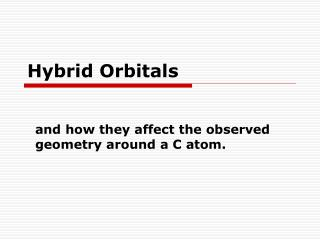# Hybrid Orbitals - PowerPoint PPT PresentationDownload PresentationHybrid Orbitals

Hybrid OrbitalsDownload Presentation## Hybrid Orbitals

- - - - - - - - - - - - - - - - - - - - - - - - - - - E N D - - - - - - - - - - - - - - - - - - - - - - - - - - -
##### Presentation Transcript

1. Hybrid Orbitals and how they affect the observed geometry around a C atom.

2. Valence Electrons • Valence electrons are usually the s and p electrons in the outermost unfilled shell of an atom. • For main group elements, the number of valence electrons is the same as the group number. • Carbon is in group IV and has four valence electrons.

3. Valence Electrons • Another way to find the valence electrons is to determine the electronic configuration of the atom. • Carbon has 6 electrons. The electrons fill the lowest energy orbital first and work their way up (the aufbau principle). • You might remember the orbital energy diagram (next slide). • C: 1s22s22p2

4. Orbital Energy Diagram for Carbon 4p 4s 3d 3s 3p ENERGY 2p 2s 1s

5. Valence Electrons • Since for tests you are not given an orbital energy diagram but you are given a periodic table, be able to use the periodic table to determine an electronic configuration (next slide). • C: 1s22s22p2

6. Starting at the top left (1s), put in all 6 of C’s electrons. 1 C: 1s22s22p2 2 3 4 5 6

7. Orbital Shapes • s orbitals are spherical. y x z

8. Orbital Shapes • p orbitals have two lobes. There are three p orbitals and they are perpendicular to each other.

9. Valence Bond Theory • Valence bond theory says that bonds between atoms are formed by the overlap of atomic orbitals. • If we use the 2s and 2p orbitals of C to explain its bonding in methane (CH4), we would predict 90° bond angles. • The bond angles are 109.5°.

10. VSEPR Theory • Valence shell electron pair repulsion (VSEPR) theory says that the geometry around an atom is determined by the number of its electron domains and the fact they want to be as far from each other as possible. • 4 electron domains, tetrahedral geometry, 109.5° bond angles

11. sp3 Hybrid Orbitals Energy is used to promote one 2s e- to the empty 2p orbital. 2p 2s Orbital energy Carbon has four e- available for bonding. However, the orbitals containing the four e- must first be mixed to form four orbitals with the same energy. 1s

12. sp3 Hybrid Orbitals sp3 Orbital energy Mixing the four orbitals gives carbon four sp3 hybrid orbitals with the same energy. 1s Tetrahedral VSEPR geometry  sp3 hybrid orbitals.

13. sp3 Hybrid Orbitals

14. Valence Bond Theory and Double Bonds • In ethylene, there are three electron domains around each C atom. VSEPR theory says the geometry is trigonal planar and the bond angles are 120°. • A different set of hybrid orbitals must be present. They are formed from the 2s orbital and two of the 2p orbitals.

15. sp2 Hybrid Orbitals Ethylene is C2H4 and has a Lewis structure of: All of the atoms of ethylene lie in a single plane. Bond angles are 120°. H H H H C = C One 2p orbital remains unhybridized. 2p sp2 E 1s orbital energy diagram for C Trigonal planar VSEPR geometry  sp2 hybrid orbitals.

16. sp2 Hybrid Orbitals

17. Valence Bond Theory and Double Bonds H H H H Ethylene C = C σ bonds are formed from the end-to-end overlap of orbitals. • Each C – H bond is a σ bond formed from the overlap of a C sp2 orbital with the H 1s orbital. • One of the C – C bonds is a σ bond formed from the overlap of C sp2 orbitals.

18. Valence Bond Theory and Double Bonds H H H H Ethylene C = C 3. The second C – C bond is a π bond formed from the overlap of the unhybridized C 2p orbitals.

19. π Bonds H H H H Ethylene C = C The overlap in a π bond is side-to-side (NOT end-to-end like in σ bonds).

20. Valence Bond Theory and Triple Bonds • In acetylene, there are two electron domains around each C atom. VSEPR theory says the geometry is linear and the bond angles are 180°. • A third set of hybrid orbitals must be present. They are formed from the 2s orbital and one of the 2p orbitals.

21. sp Hybrid Orbitals Acetylene is C2H2 and has a Lewis structure of: H - C ≡ C - H All of the atoms of acetylene lie in a single line. Two 2p orbitals remain unhybridized. 2p E sp 1s orbital energy diagram for C Linear VSEPR geometry  sp hybrid orbitals.

22. sp Hybrid Orbitals

23. Valence Bond Theory and Triple Bonds Acetylene H - C ≡ C - H 1. Each C – H bond is a σ bond formed from the overlap of a C sp orbital with the H 1s orbital. 2. One of the C – C bonds is a σ bond formed from the overlap of C sp orbitals.

24. Valence Bond Theory and Triple Bonds Acetylene H - C ≡ C - H 3. The other two bonds are π bonds formed from the overlap of the C 2p orbitals.

25. Summary